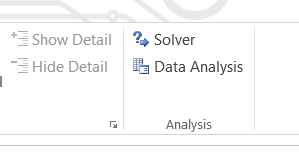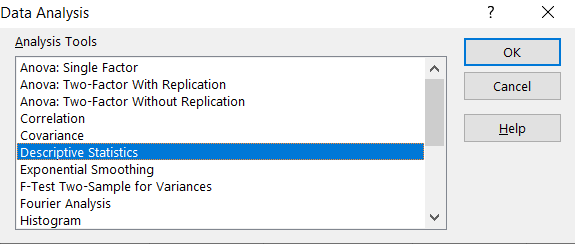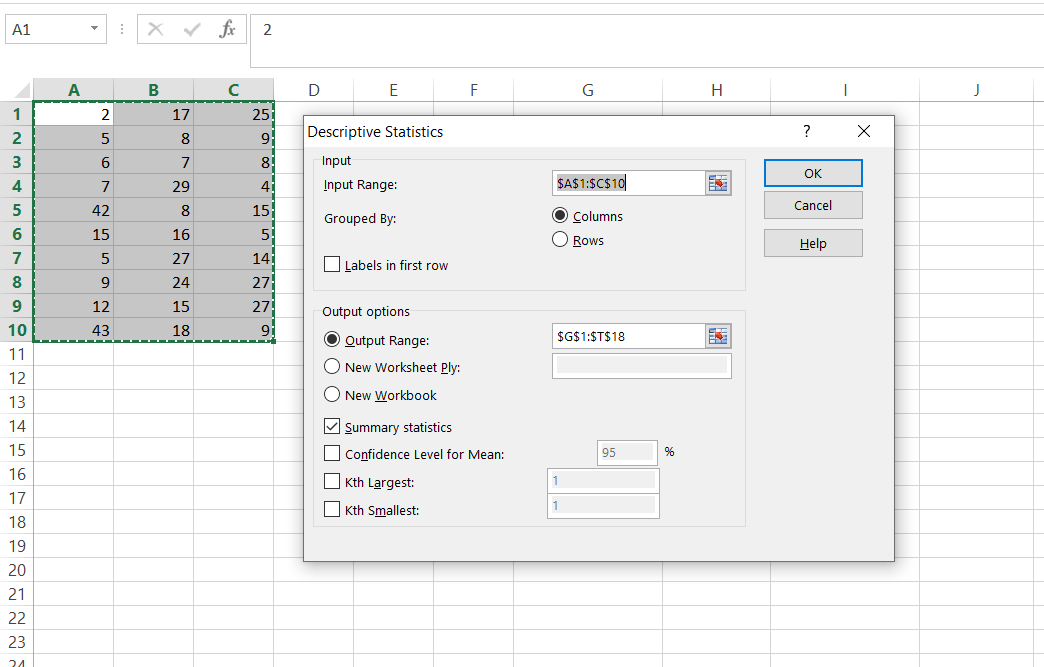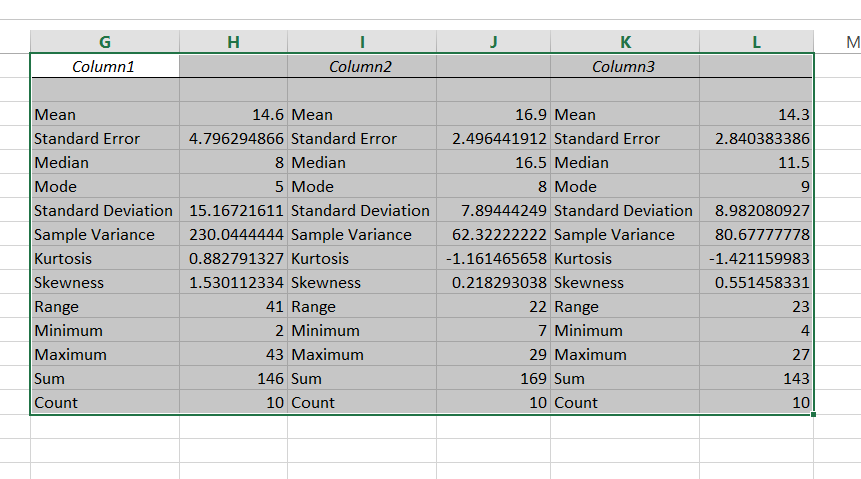#### How to calculate variance using the Data Analysis Toolpak Add-In?

Variance is a measure of how spread out the values are in a dataset. It is calculated by averaging the squared deviations from the mean. A higher variance indicates greater variability among the values, while a lower variance indicates less variability.

There is also a possibility to calculate the Variance without using an Excel formula. Instead of the Var.S formula, you will use an Excel add-in.

## The Data Analysis Toolpak Add-In

The Data Analysis Toolpak Add-In is a free add-in that can be installed in Excel. It provides a number of statistical functions, including the ability to calculate variance.

## Benefits of Using the Data Analysis Toolpak Add-In

There are several benefits to using the Data Analysis Toolpak Add-In to calculate variance.

• The Data Analysis Toolpak Add-In can calculate variance for a larger dataset than the VAR function. The VAR function can only calculate variance for a dataset of up to 32,767 values, while the Data Analysis Toolpak Add-In can calculate variance for any size dataset.
• The Data Analysis Toolpak Add-In can calculate variance for non-numeric data. The VAR function can only calculate variance for numeric data, but the Data Analysis Toolpak Add-In can calculate variance for text and date data as well.
• The Data Analysis Toolpak Add-In provides more statistical information than the VAR function. The VAR function only returns the variance, but the Data Analysis Toolpak Add-In also returns the mean, standard deviation, minimum value, maximum value, and other statistical measures.

## Calculating Variance using the Data Analysis Toolpak Add-In

Navigate to the Data Tab on the Ribbon. Click the Data Analysis button.A new window appears. Choose Descriptive Statistics and click OK.Enter the proper parameters:

• Input Range: range of your data (\$A\$1:\$C\$10 in my example)
• Labels in first row: there are no labels in my data table.
• Output Range: choose where you want to get your variance calculated (I chose the same Sheet \$G\$1:\$T\$18)
• Summary statistics: make sure it is checkedAnd this is how you can see my Statistics summary. Sample variance is calculated in row 8.The Data Analysis Toolpak Add-In is a powerful tool that can be used to calculate variance and other statistical measures for your data. It is easy to use and can handle larger datasets than the VAR function. If you need to calculate variance for your data, the Data Analysis Toolpak Add-In is a great option.